## Example Questions

2 Next →

### Example Question #11 : Simplifying Polynomials

Which of the following is equivalent to the expression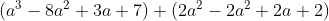?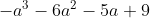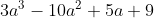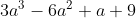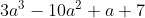Explanation:

A look at the answer choices tells you that you need to simplify the expression to arrive at fewer terms. To simplify this expression, you need to remove the parentheses and combine like terms. Fortunately, the process is to add the two parentheses and there are no coefficients or negatives between them, so you can simply lift the parentheses entirely and then combine.  That leaves you with:2 Next →

### All SAT Mathematics Resources